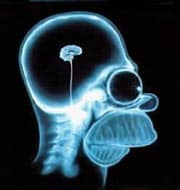# Science without sense…double nonsense

### Píldoras sobre medicina basada en pruebas

Posts tagged Statistical

## Size does matter

Of course, we talk about samples.

For various reasons, scientific studies often use samples drawn from a population on which you want to get any specific conclusion. This sample will have to be selected so that it faithfully represents the population from which it is extracted but, how large sample ought to be: big or small?. Well, neither one thing nor the other: the sample must be of the appropriate size.

We’d need to rest a bit after reasoning until getting this conclusion but, first, we’ll try to find out what problems too large or too small samples can cause us.

Drawbacks of larger than needed samples are obvious: greater expenditure of time and resources.

Moreover, we already know that sometimes it is enough to increase sample size in order to obtain statistical significance, although we also know that if we use this technique in excess we can obtain significance among differences that are too small and, although the difference may be real, its clinical relevance can be limited. Doing that way we expend time and energy (and money) and can also create confusion about the importance of the difference found. So, as in many other aspects of life and medicine, when we speak about samples, more is not always better (nor it is better to have it larger).

What if the sample is small?. It’s a bit the opposite situation. The smaller the sample, the greater the imprecision (the wider the confidence intervals). As a result, differences have to be larger to reach statistical significance. We thus run the risk that, although there’s a real difference, we won’t be able to assure its existence due to the too small sample, losing the opportunity to shown differences that, although small, could be clinically relevant.

It’s clear, therefore, that the sample size must be the appropriate size and that, to avoid more evil, we should calculate it before doing the study.

Formulas to come up with the sample size depend on the statistical we are measuring and on the fact of whether we are estimating a population (a mean, for example) or we want to do a hypothesis testing between two variables or samples (to compare to samples, two proportions, etc.). In any case, most statistical programs are capable to calculate it quickly and without flinching. We just have to set the values of three parameters: the type 1 error, the study power and the minimum clinically relevant difference.

Type 1 error is the probability of rejecting the null hypothesis being true, so concluding that there’s a difference that is, indeed, not real. It is generally accepted that this probability, which is called alpha, must be less than 5% ant it is the level of statistical significance usually employed in hypothesis testing.

Type 2 error is the probability of concluding that there’s no difference when in fact there is (and not to reject the null hypothesis). This value is known as beta and a minimum of 80% is generally accepted as an appropriate level. Its complementary value (1-beta, or 100-beta if we like more percentages values) is what we call the power of the study.

Finally, the minimal clinically relevant difference is that that the study is able to detect, given that it actually exists. This value is set by the researcher according to the clinical context and has nothing to do with the statistical significance of the study.

Using these three parameters, we’ll calculate the required sample size to detect the difference considered relevant from the clinical point of view and with the desired amount of error.

Sometimes we can do this reasoning in the reverse way. If our sample size has an upper limit, whatever the reason, we can estimate the difference we’ll be able to detect before doing the study. If this difference is less than the clinically relevant difference, better save our work, because we’ll be at risk of not reach a conclusion because of the small and misleading sample, getting the conclusion that a real difference that not exists. Similarly, if we must stop the study before its programmed ending we should calculate if the achieved sample gives us enough power to discriminate the level of difference we proposed at the beginning of the study.

According to the variable we are measuring, sometimes we’ll need other data such as the population’s mean or standard deviation to estimate the sample size. If we don’t know these values we can do a pilot study with a few patients (at the judgment of the researcher) and calculate the sample size with the preliminary results.

One last thought before going to cool our heads. The sample size is calculated to estimate the primary outcome, but we cannot be sure our sample is appropriate to estimate any other parameters we measure during the study. Very often, we can see trials very well design to show the effectiveness of a treatment but incapable of provide conclusive data about its safety. But that’s another story…